## Introduction

A little over a year ago, I did this project: https://github.com/yiransheng/hn-activities-in-bq

To summarize, it’s basically a cron job (running on webtask) which queries HN api for certain front page story stats twice an hour. Results are streamed into Google BigQuery. The README over there provides a little bit more information and simple guides if you want to run it yourself.

Since then, this little script (although I had quite a bit of trouble to shrink down the size of its deployed version, due to bloated Google npm packages) has been running over a year. The BigQuery table currently sits at 30 something MB! Quite some “big” data befitting its storage. Jokes aside, hourly stats of every 30 frontpage stories over an year is actually a meaningful dataset to run some fun analysis on - something this post aims to be a first of.

I had hacked together a Jupyter Notebook back then, visualizing a few hours worth of data. If you want get a quick look at the dataset, I suggest head over there (or stay here, as we are getting into it right afterwards).

By the way, this is an RMarkdown notebook, and let’s start by importing some essential packages:

```library(tidyverse)

library(tsibble)

library(ggthemes)

library(broom)

library(pander)
```

## The Dataset

I have exported the BigQuery table as csv, a quick look at this file:

```wc -l bq-hnactivity.stats.csv
```
``## 314653 bq-hnactivity.stats.csv``

Almost a third of a million rows, not too shabby. Reading it into a dataframe, while specifying all column types:

```interval.format <- "%F %H:%M:%OS UTC"

created.format <- "%F %H:%M:%S UTC"

locale = locale(tz='UTC'),

col_types=cols(

story_id            = col_character(),

story_createdat     = col_datetime(format=created.format),

story_point_begin   = col_integer(),

story_point_delta   = col_integer(),

interval_begin      = col_datetime(format=interval.format),

interval_end        = col_datetime(format=interval.format),

comment_count_begin = col_integer(),

comment_count_delta = col_integer(),

story_rank_begin    = col_integer(),

story_rank_end      = col_integer()

))

hnstats\$interval_duration <-

difftime(hnstats\$interval_end, hnstats\$interval_begin)
```

What is this `_begin` and `_delta` business? To quote how I wrote it back then:

Every hour (or determined by other CRON configurations) the script takes two 15-seconds-apart snapshots of Hacker News frontpage statistics (top 25 stories and their rank, story point and comment count)

The basic idea is trying to capture an upvote or a comment as it happens and reflect it in the dataset. To truly analyze this type of realtime traffic, ideally, I should perform these quries every 15 seconds. Well, I didn’t want to bankrrupt myself… therefore this slight addition of 15-seconds apart snapshots on an the much less frequent hourly jobs.

### Clean Up

The dataset is mostly clean, the webtask script handles errors as strictly as it could, and makes sure no `NA` / `NaN` values are committed to BigQuery. However there is a small issue within the dataset, in terms of `interval_duration` column (time difference between two snapshot queries):

```pander(summary(

as.numeric(hnstats\$interval_duration, units = "secs")

))
```
Min. 1st Qu. Median Mean 3rd Qu. Max.
10.01 15 15 15 15 30.03

A few rows of data that had non-15-second intervals during my testing phase made it into the dataset (I was testing out 10-second and 30-second intervals, adjusting for webtask’s timeout setting). Here we filter these out, and make sure all remaining intervals are within ms of 15 seconds.

```INTERVAL_DURATION <- as.difftime(15, units = "secs")

ε.interval_duration <- as.difftime(0.01, units = "secs")

hnstats %>% filter(

interval_duration >= INTERVAL_DURATION - ε.interval_duration &

interval_duration <= INTERVAL_DURATION + ε.interval_duration

) -> hnstats

hnstats
```

## Lifecycle of a Popular Story

To warm up, we will follow a single popular story through its lifetime on HN front page, and visualize how it garnered upvotes and climbed ranks. To do this, let’s quickly collect top 10 stories by their number of occurrences in the dataset:

```story_ids <- hnstats %>%

group_by(story_id) %>%

summarize(n = n()) %>%

top_n(10, n) %>%

arrange(-n)

story_ids\$story_id,

sep = ""

)

pander(story_ids)
```
16775093 55 https://news.ycombinator.com/item?id=16775093
16775744 51 https://news.ycombinator.com/item?id=16775744
16990495 51 https://news.ycombinator.com/item?id=16990495
15314006 50 https://news.ycombinator.com/item?id=15314006
15367531 50 https://news.ycombinator.com/item?id=15367531
15772065 49 https://news.ycombinator.com/item?id=15772065
16134618 49 https://news.ycombinator.com/item?id=16134618
17993933 49 https://news.ycombinator.com/item?id=17993933
15752022 48 https://news.ycombinator.com/item?id=15752022
15466599 47 https://news.ycombinator.com/item?id=15466599
15723926 47 https://news.ycombinator.com/item?id=15723926

Story 16775093 was seen 55 times - roughly 2 days on the front page, an eternity on this high-traffic site.

Plotting its rank over time:

```hnstats %>%

filter(story_id == story_ids\$story_id) %>%

select(

interval_begin,

story_createdat,

story_point_begin,

comment_count_begin,

story_rank_begin

) %>%

arrange(interval_begin) -> single_story

single_story %>%

ggplot(., aes(

x = as.numeric(interval_begin - story_createdat, units = "hours"),

y = factor(story_rank_begin, levels = c(30:1)),

group = 1

)) +

geom_line(stat = "identity") +

geom_point(size = 2) +

ylab("Story Rank") +

xlab("Story age (hours)") +

theme_tufte(base_size = 15)
```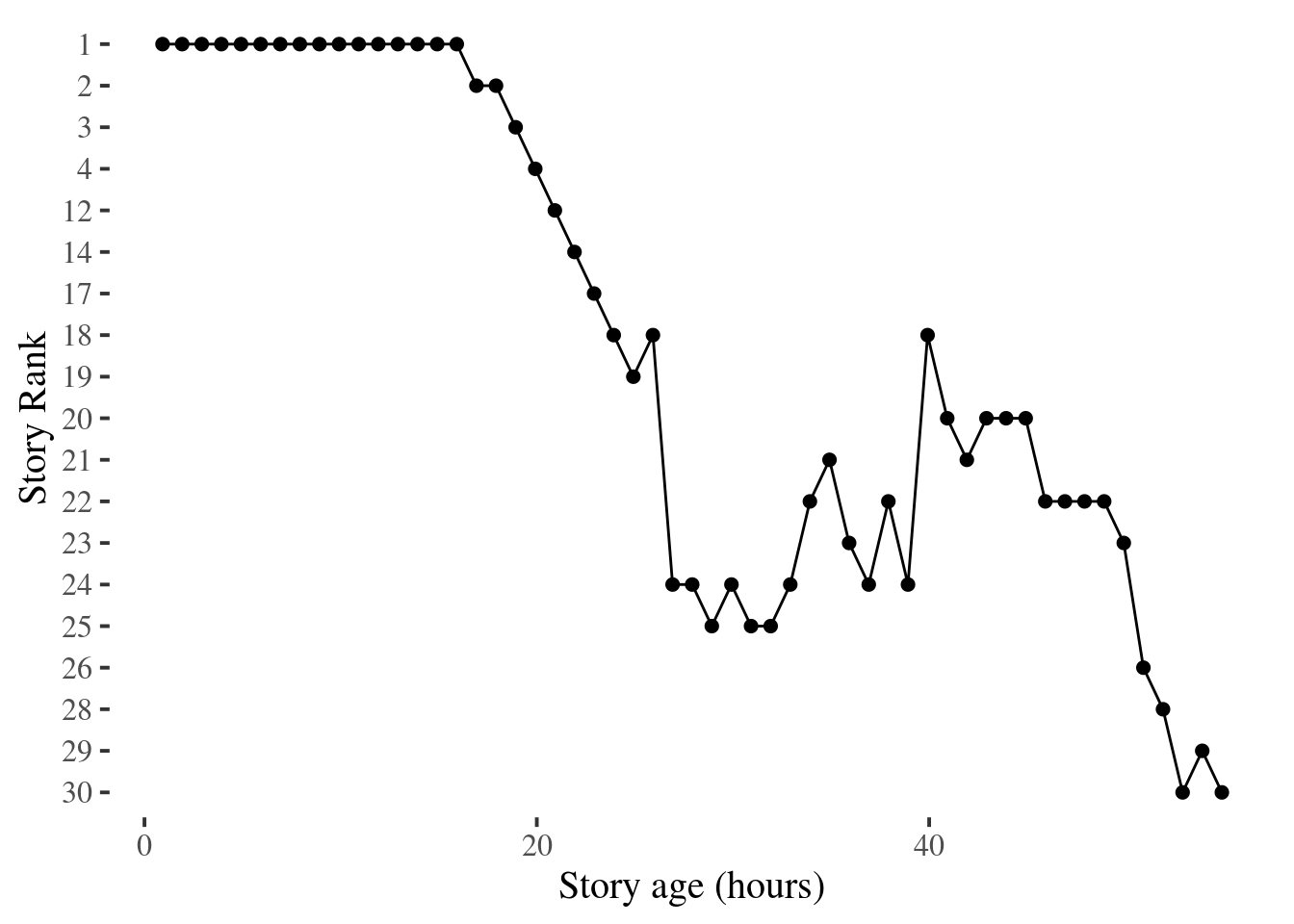Note the first time this story appears in the dataset, it had already been crowned the top spot (rank 1). Most likely, it graduated the `new` category onto frontpage within an hour of its submission (barring any data collection errors causing my script to miss it). It remained as number-one story for close to 20 hours before dropping in rank steadily. Just around a full 24 hours later after its first frontpage appearance (when most stories retire from frontpage), it jumped back from bottom of the page to the middle, and enjoyed roughly another day of exposure, before finally fading away. We will see this “second wind” pattern again on an aggregate level later.

Additionally, here’s its comment count and story points over time:

```single_story %>%

gather(metric, value, story_point_begin:comment_count_begin) %>%

ggplot(., aes(

as.numeric(interval_begin - story_createdat, units = "hours"), value

)) +

facet_wrap(~metric) +

geom_line() +

geom_point(size = 1) +

xlab("Story age (hours)") +

theme_tufte(base_size = 15)
```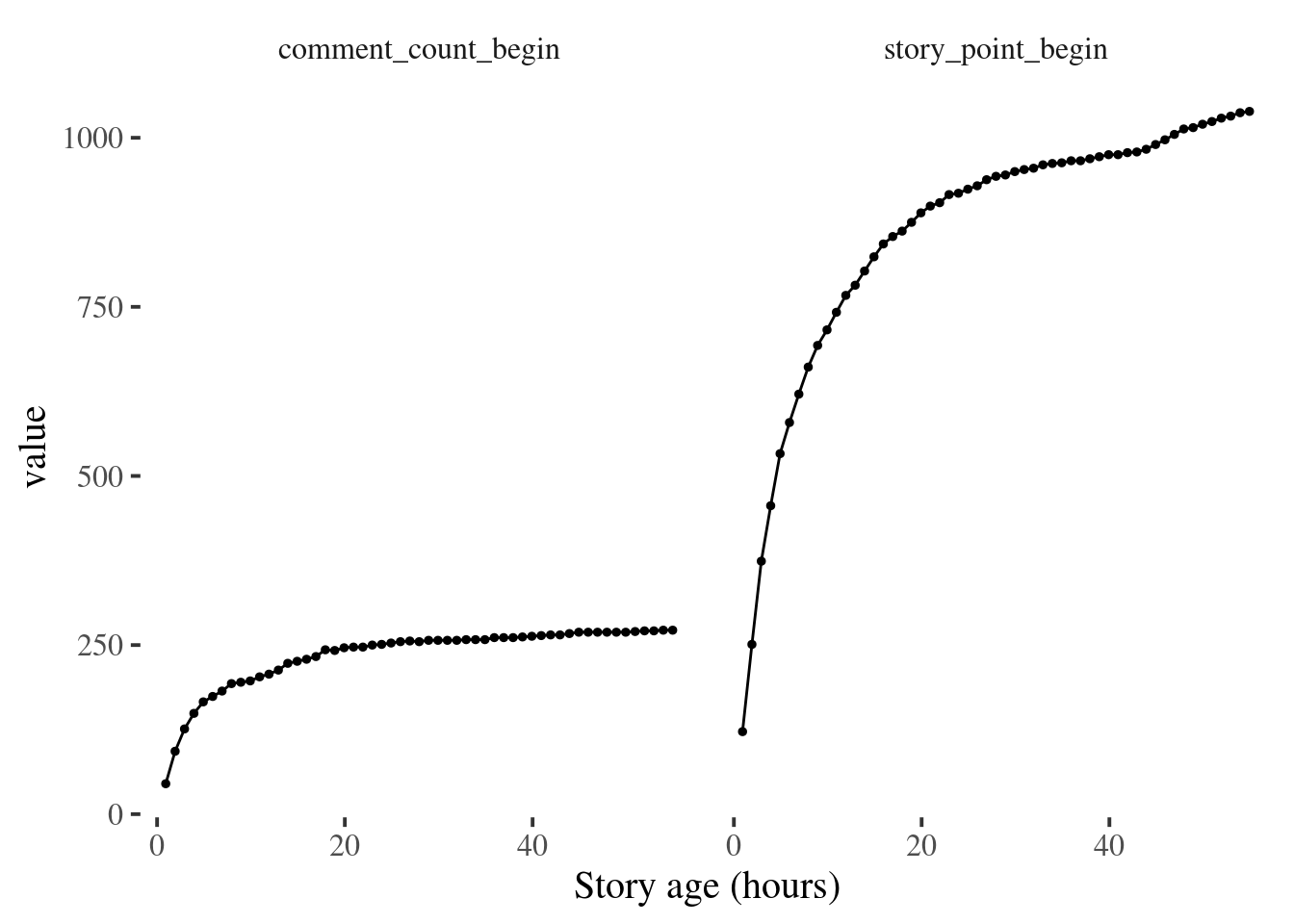## Heatmapping Front Page Activities

Now, let’s use the collected delta metrics on comments and upvotes. This section is mostly visualizing obvious patterns we would expect from a high-traffic news list. To begin with, a tally of observed comments and upvotes (for better contrast shading is done on log scale).

```hnstats %>%

ggplot(., aes(factor(story_point_delta), factor(comment_count_delta))) +

geom_tile(stat = "bin_2d", color = "#c0c0c0") +

scale_fill_gradient(low = "white", high = "#595959", trans = "log10") +

xlab("story_point_delta") +

ylab("comment_count_delta") +

theme_tufte(base_size = 15)
```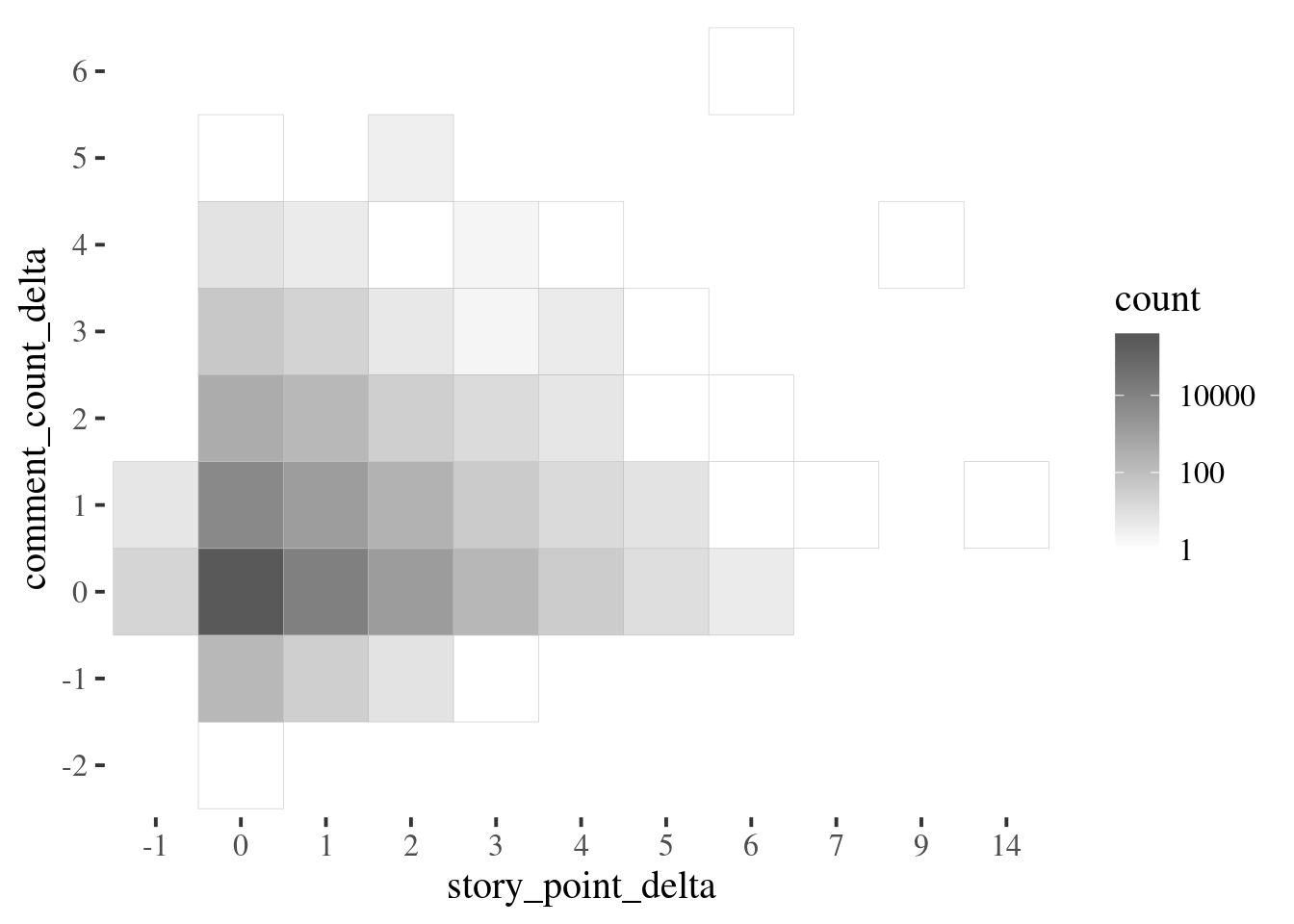As expected, most common group is the tile where the sampling script failed to capture any metric changes.

Next, we will focus on a subset with positive `story_point_delta` captured in their 15-second interval - and plot a heatmap of upvotes by rank (this time using linear shading scale). This offers a nice statistical picture of front page upvotes.

```hnstats %>%

filter(story_point_delta > 0) %>%

ggplot(., aes(factor(story_point_delta), factor(story_rank_begin, levels = seq(30, 1, -1)))) +

geom_tile(stat = "bin_2d", color = "#c0c0c0") +

scale_fill_gradient(low = "white", high = "#595959") +

ylab("Story Rank") +

theme_tufte(base_size = 15)
```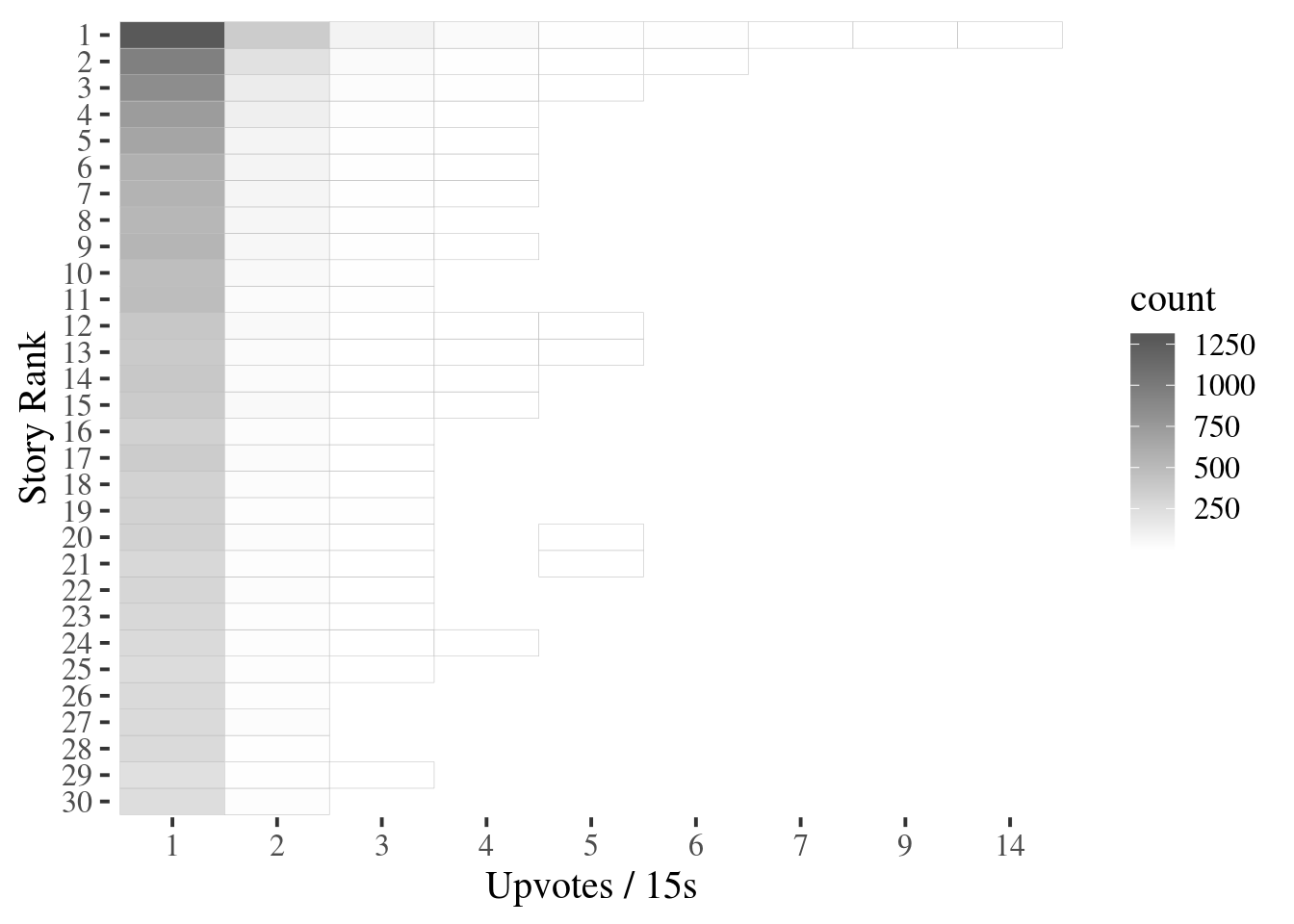A similar pattern manifests itself for comments:

```hnstats %>%

filter(comment_count_delta != 0) %>%

ggplot(., aes(factor(comment_count_delta), factor(story_rank_begin, levels=seq(30, 1, -1)))) +

geom_tile(stat="bin_2d", color="#c0c0c0") +

scale_fill_gradient(low = "white", high = "#595959") +

ylab("Story Rank") +

theme_tufte(base_size = 15)
```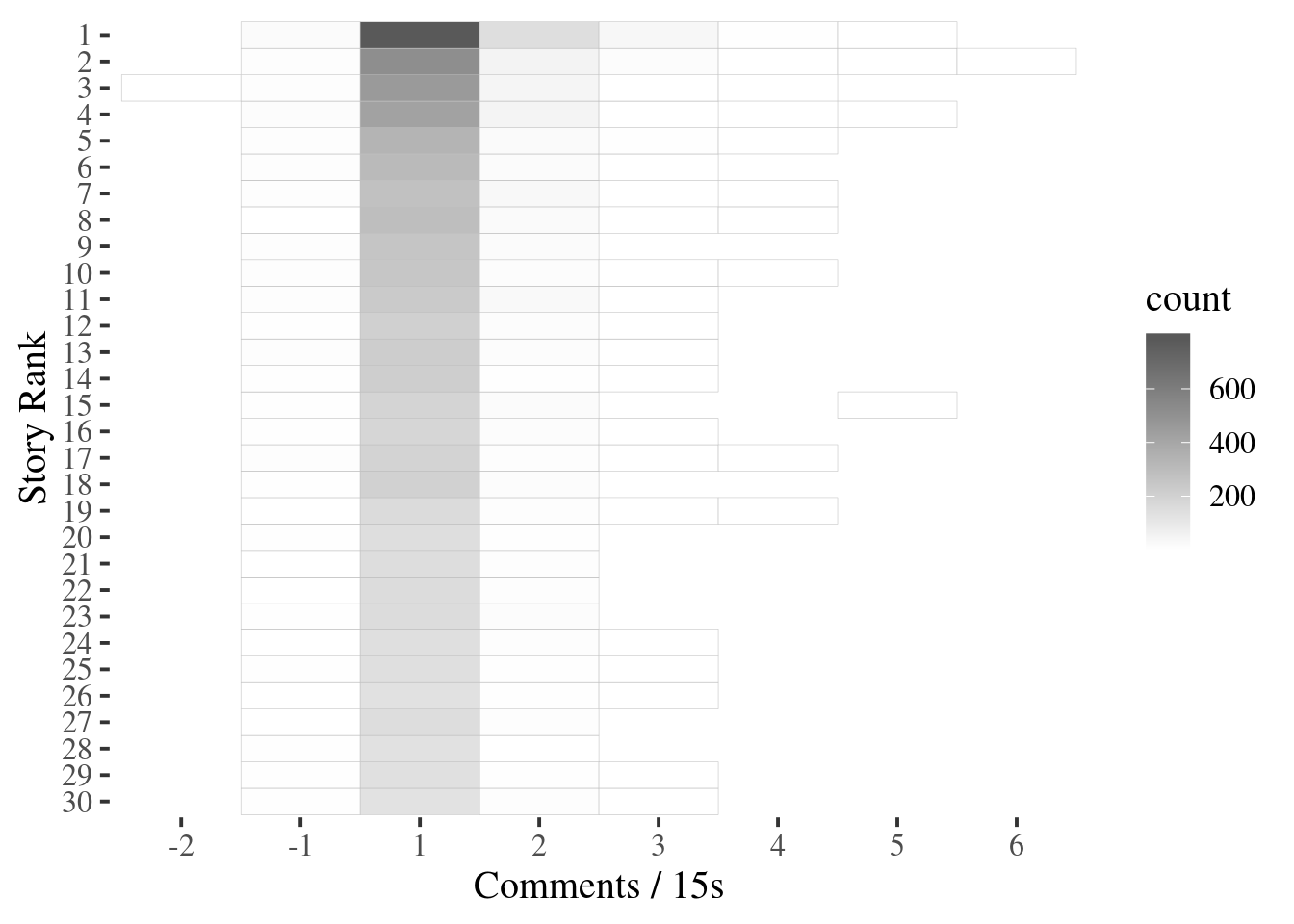## Best Submission Hours

It won’t be a proper HN analysis without answering the dire question of when the best time is to post to HN. So let’s take a stab at it. The dataset is grouped by `story_id` below, and we record the timestamp a given story is first and last seen on the front page.

```stories <- hnstats %>%

group_by(story_id) %>%

summarize(

first_seen = min(interval_begin),

last_seen = max(interval_begin),

created_at = median(story_createdat)

) %>%

mutate(frontpage_duration = last_seen - first_seen)
```

Keep in mind, the computed `frontpage_duration` column is just an estimation. As data is only collected hourly, any story lasted less than an hour will be have an 0 value for this field. We do not have the exact time stamp when a story pops up and dropps off the frontpage. With that said, it’s useful to examine the distribution of `frontpage_duration`anyway:

```stories %>%

ggplot(., aes(x = as.numeric(frontpage_duration, units = "hours"))) +

geom_histogram(binwidth = 0.5) +

xlab("Front page duration (hours)") +

theme_tufte(base_size = 15)
```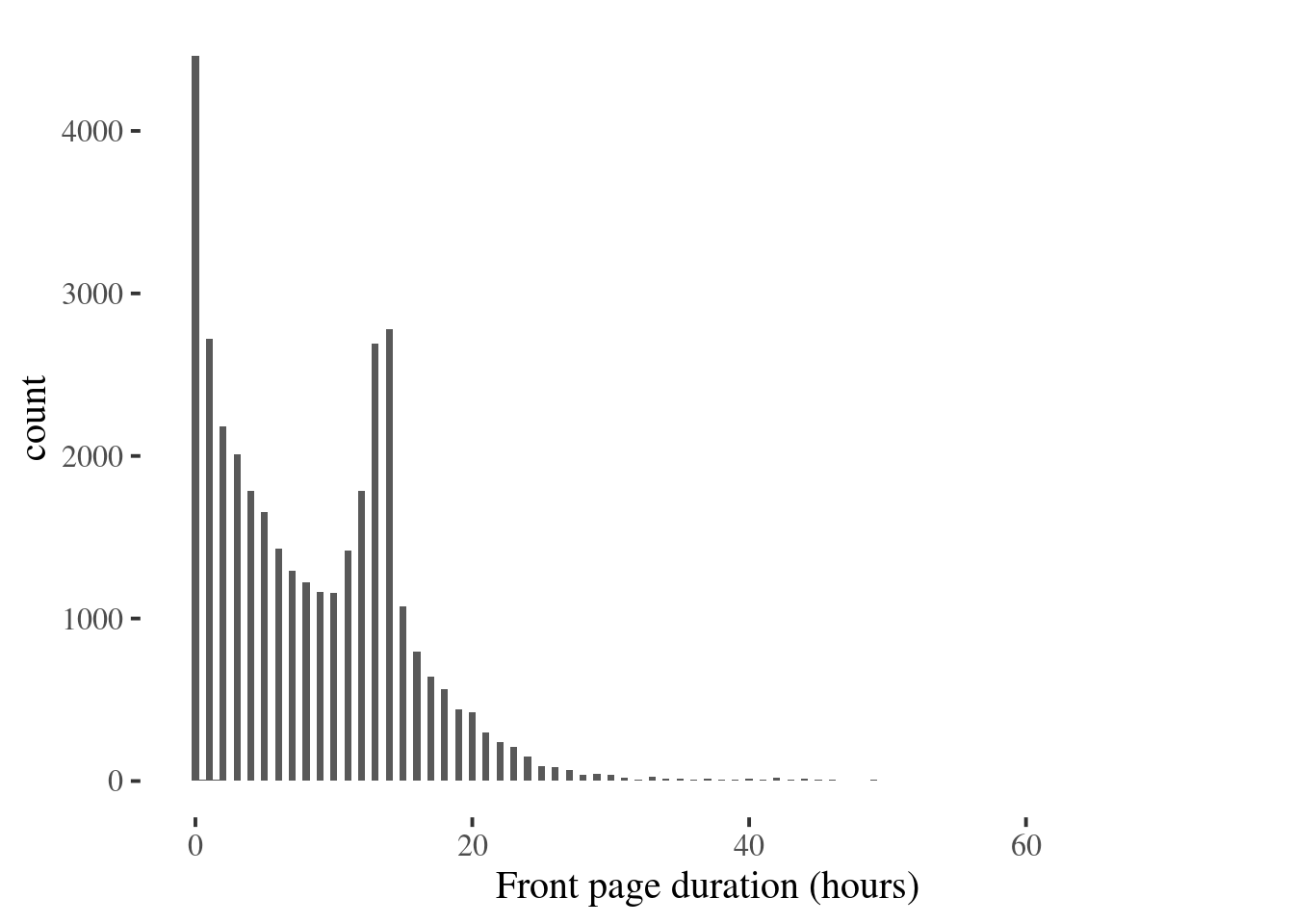Interestingly, there is a bimodal pattern here. A interpretation can be made here for the “second wind” effect. Front page stories exist in two clusters, some dropped off after no more than 20 hours, but another significant portion remained there a bit longer. The 12 hour mark seems to be the separation point - something I suspect is determined by internal HN rank algorithm.

Now onto the main task. By design, all stories in this dataset are front page stories. Therefore, to simply group them by hour of creation tells us which hour-of-day contributed the most to the front page entries. We make a quick function to plot this information, for parametrized timezones.

```best_submission_hour <- function(tz, xlab, ylab) {

hour_fmt <- "%H"

df <- stories %>%

mutate(

created_hour = strftime(created_at, hour_fmt, tz = tz)

) %>%

group_by(created_hour) %>%

summarise(count = n())

df\$created_hour <- as.POSIXct(df\$created_hour, format = hour_fmt, tz = tz)

ggplot(df, aes(created_hour, count, fill = count)) +

scale_x_datetime(date_labels = "%I:%M %p") +

scale_fill_gradient(low = "#595959", high = "#ee5959", guide = FALSE) +

geom_bar(stat = "identity") +

xlab(xlab) + ylab(ylab) +

theme_tufte(base_size = 15)

}
```

For UTC:

```best_submission_hour('UTC', 'Submission hour (UTC Time)', "Count")
```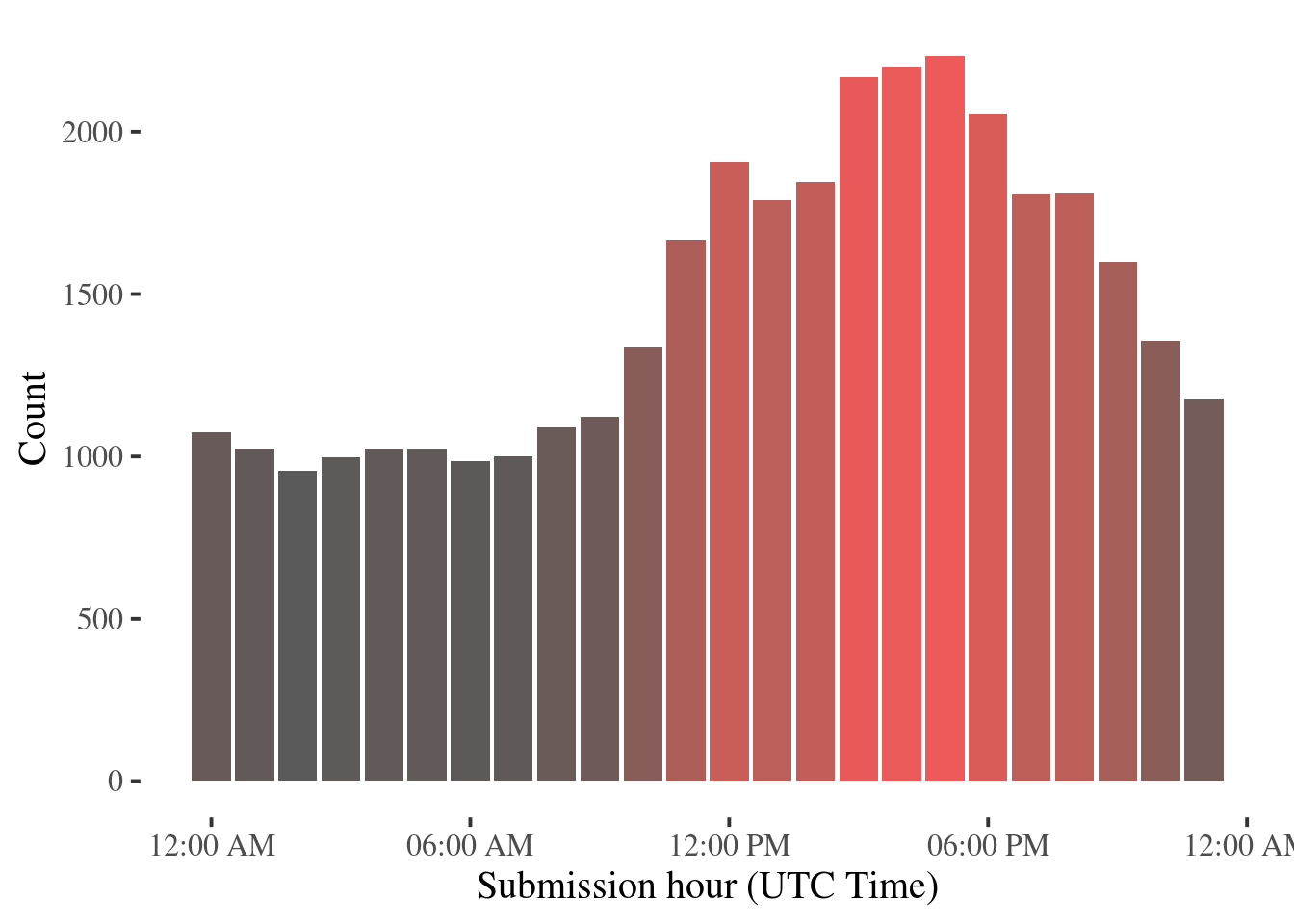This seems to be consistent with some previous findings on this topic. For example, to quote this article,

…each hour of the day (UTC). We can see that, on average, between 5 PM and 6 PM was the most active hour of the day.

And the same plot for `America/Los_Angeles` timezone, where a large portion of the HN audience resides. Note this is not a simple shift of previous plot, due to day light saving time.

```best_submission_hour('America/Los_Angeles', 'Submission hour (California Time)', "Count")
```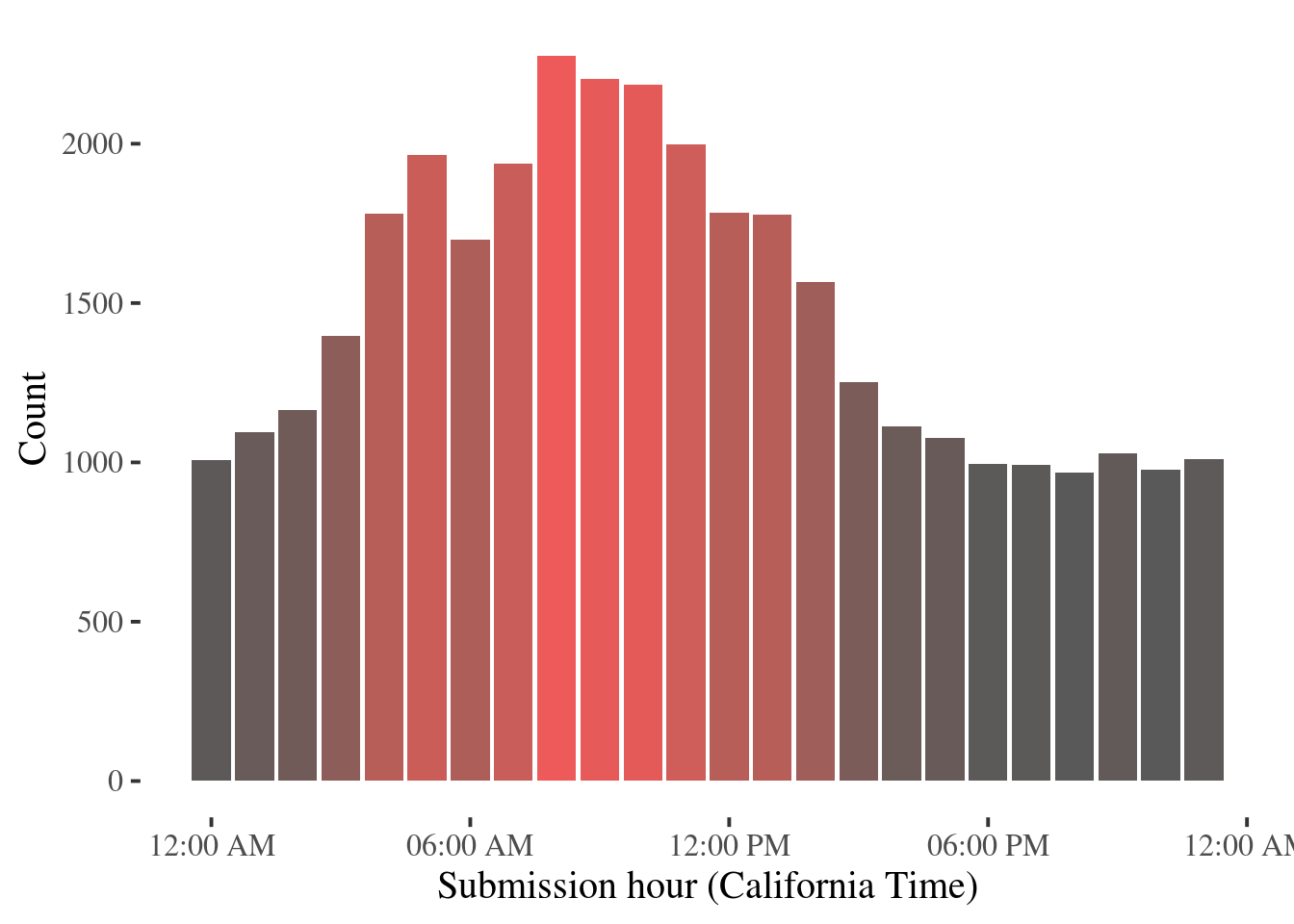Pause for a second, have you noticed something problematic here though? Yeah, that’s right - a thread of Survivor Bias sneaked in! This dataset consists only of front page stories, we have no clue about the submission volume by hour. For instance, around UTC 6:00 PM, could simply be when most submissions are made - thus producing the largest amount of front page stories. To get a sense of what time of day a random submission has the highest chance of making it to the front page, this dataset by itself is definitely not enough.

Fortunately, an estimation can be make by leveraging the public Hacker News Dataset (`bigquery-public-data.hacker_news.stories` on Google BigQuery). It is cutoff at 2015 era though. By using information from it, we are assuming the submission volume pattern with respect to hour of day has not changed significantly since then. To produce the data we need, this SQL query is used (and exported as a csv file):

```SELECT

EXTRACT(hour

FROM

time_ts AT TIME ZONE "UTC") AS time_of_day,

COUNT(DISTINCT(id)) AS submission_count

FROM

`bigquery-public-data.hacker_news.stories`

GROUP BY

time_of_day
```

The same query is also peformed for `America/Los_Angeles` timezone, here we read in both csv files.

```submissions_by_hour = read_csv("hn_submissions_by_hour.csv")
```
``````## Parsed with column specification:
## cols(
##   time_of_day = col_integer(),
##   submission_count = col_integer()
## )``````
```submissions_by_hour %>%

filter(!is.na(time_of_day)) %>%

```
``````## Parsed with column specification:
## cols(
##   ca_time_of_day = col_integer(),
##   submission_count = col_integer()
## )``````
```submissions_by_ca_hour %>%

filter(!is.na(ca_time_of_day)) %>%

```

Modifying our plot function a bit, and this time, we will plot `front page count / submission_count` on the `y` axis - a ratio hopefully would be proportion to the probability of a random submission landing on front page:

```real_best_submission_hour <- function(tz, join_with, xlab, ylab) {

hour_fmt <- '%H'

df <- stories %>%

mutate(

created_hour=strftime(created_at, hour_fmt, tz=tz)

) %>%

group_by(created_hour) %>%

summarise(count=n()) %>%

left_join(join_with, by=c("created_hour" = "hour"))

df\$created_hour=as.POSIXct(df\$created_hour, format=hour_fmt, tz=tz)

ggplot(df, aes(created_hour, count / submission_count, fill=count / submission_count)) +

scale_x_datetime(date_labels="%I:%M %p") +

scale_fill_gradient(low = "#595959", high = "#ee5959", guide=FALSE) +

geom_bar(stat="identity") +

xlab(xlab) + ylab(ylab) +

theme_tufte(base_size = 15)

}
```

For UTC:

```real_best_submission_hour('UTC', submissions_by_hour, "Submission hour (UTC Time)", "Relative Front Page Prob.")
```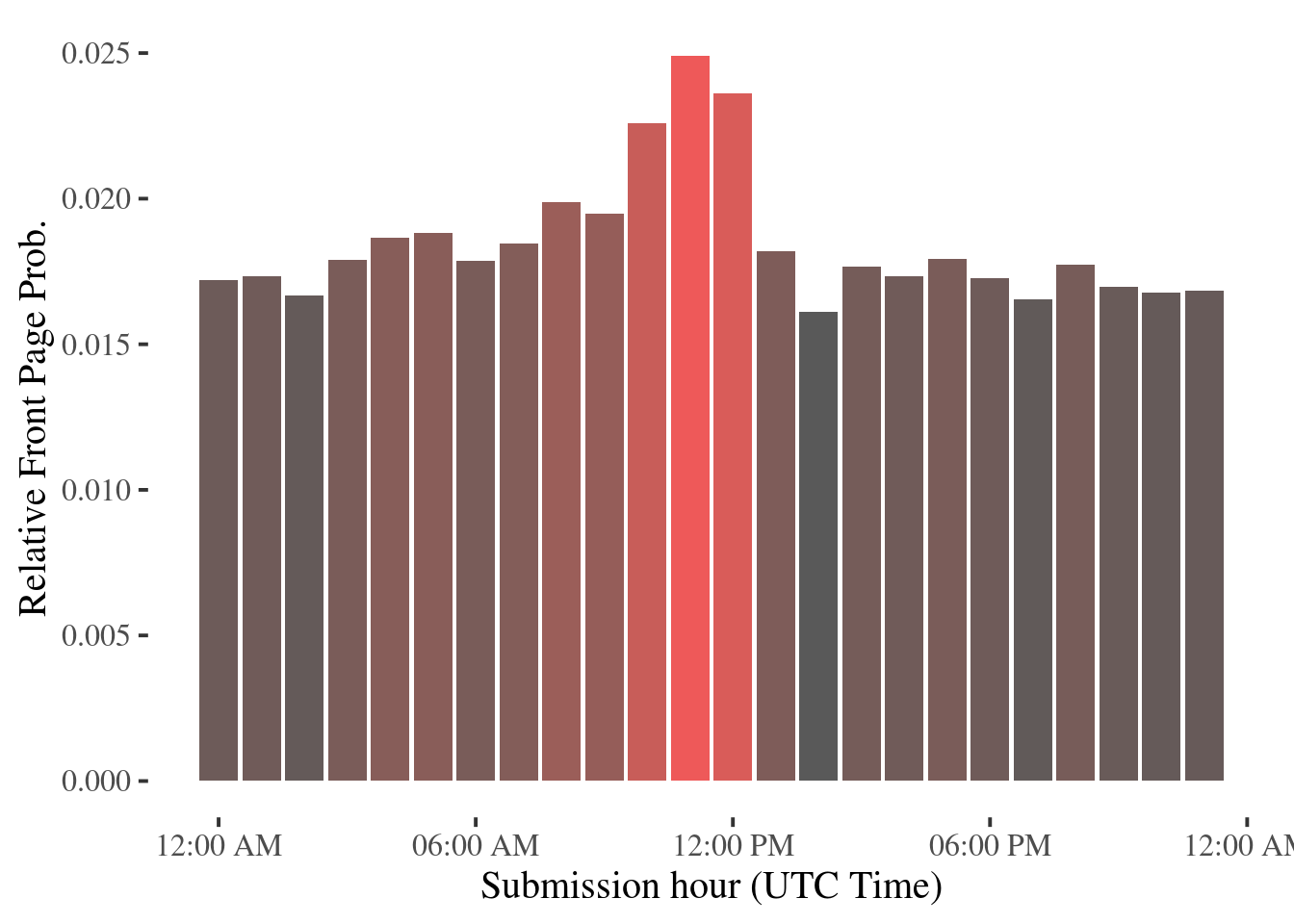For California time:

```real_best_submission_hour('America/Los_Angeles',

submissions_by_ca_hour,

'Submission hour (California Time)', "Relative Front Page Prob.")
```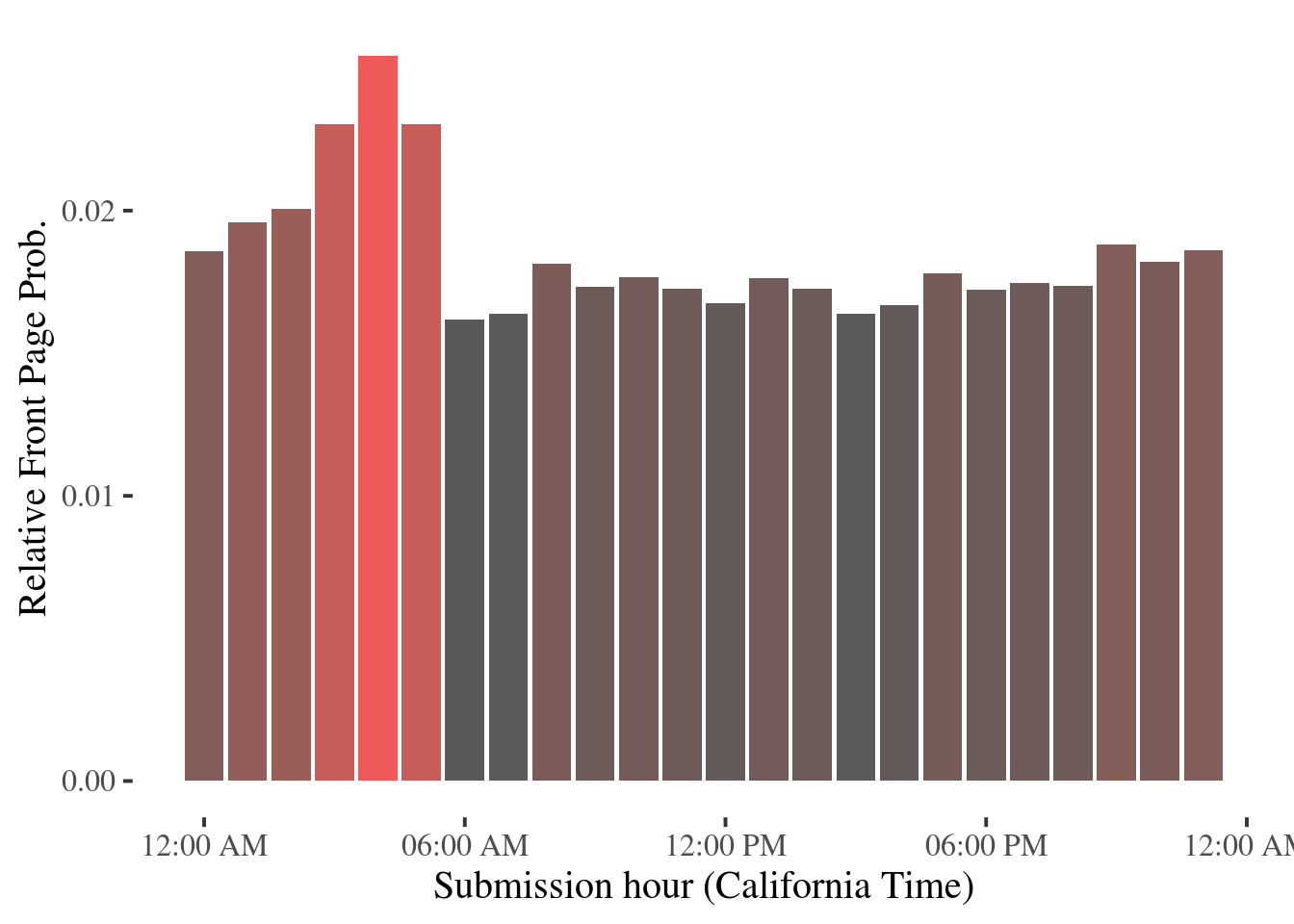Very interestingly, the “wavy” pattern from absolute front page story count plots disappeared, instead, a single narrower peak stands above an otherwise roughly uniform line. So it seems 3:00AM - 5:00AM California time gives you the best shots at the front page, when choosing when to submit. Wait until everybody else is asleep!

## Rolling Story Counts

In this section, we will take a look at another measure of front page activity levels - rolling unique story count over last 12 hours. At any given time, we do a tally of number of unique stories observed over the prior 12-hour period. Exploratory analysis so far hints at a time-of-day effect, we will keep that in mind as well.

Some data preparation, sort the dataset by time.

```hnstats %>%

arrange(interval_begin) %>%

select(story_id, interval_begin) -> story_id_by_time
```

Next, we do a rolling count distinct aggregation (or windowing in the SQL world).

```count_distinct_id <- function(ids, ts, ...) {

last_t <- max(ts)

first_t <- last_t - as.difftime(12, units = "hours")

keep <- ts >= first_t

n_distinct(ids[keep], na.rm = T)

}

# up to 30 stories per collection, and keep datums

# from the last 15 collections

WINDOW_WIDTH <- 30 * 15

story_id_by_time\$story_counts_rolling_12h <- slide2_int(

pull(story_id_by_time, story_id),

pull(story_id_by_time, interval_begin),

.f = count_distinct_id,

.size = WINDOW_WIDTH,

)

story_id_by_time %>%

group_by(interval_begin) %>%

summarise(story_counts_rolling_12h = last(story_counts_rolling_12h)) -> story_counts
```

Plot the entire year worth of data:

```story_counts %>%

ggplot(aes(x = interval_begin, y = story_counts_rolling_12h)) +

ylab("Rolling 12h Front Page Story Count") + xlab("") +

geom_line() +

theme_tufte(base_size = 15)
```
``## Warning: Removed 15 rows containing missing values (geom_path).``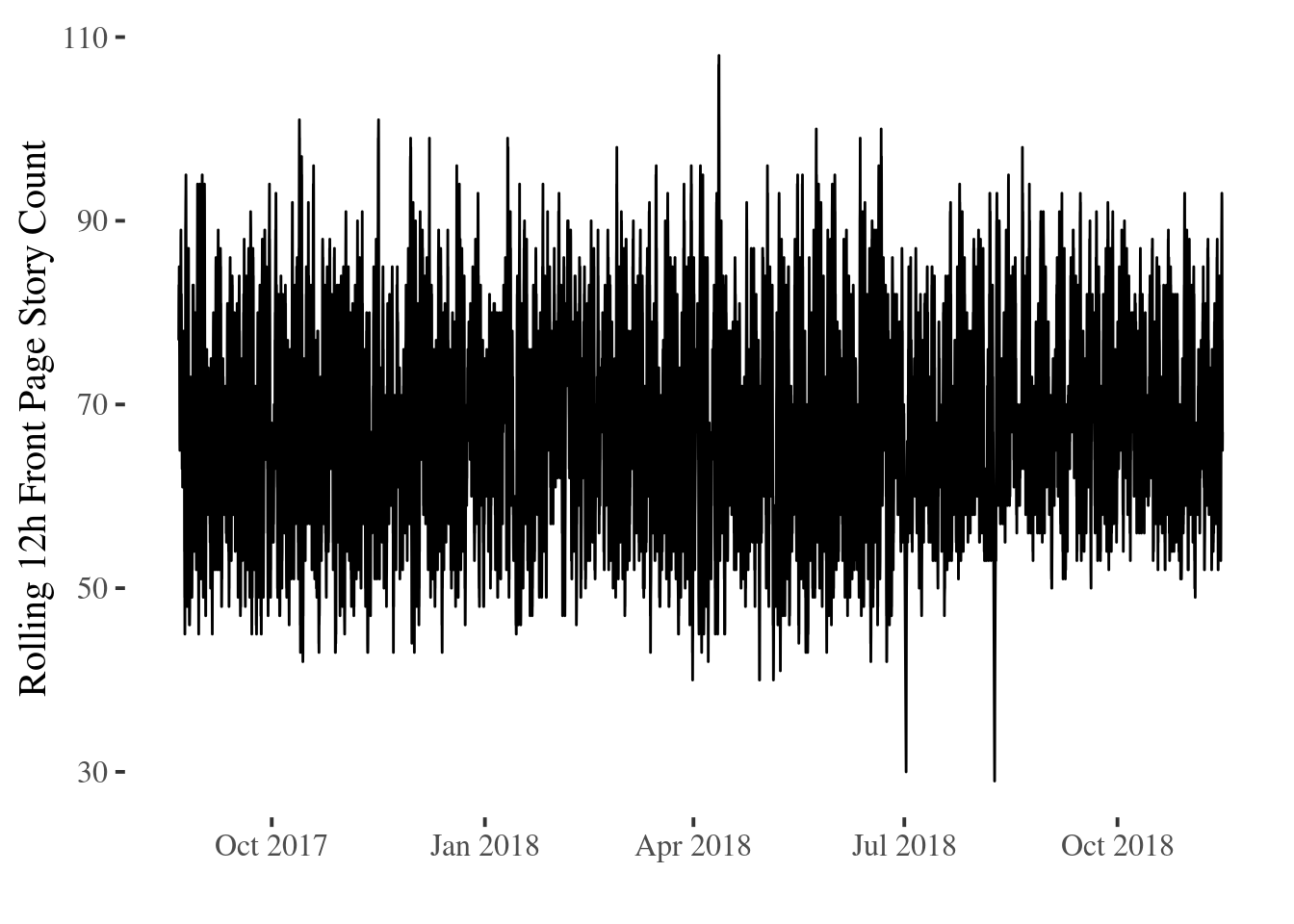Just eyeballing it, this series definitely seems stationary. The front page lists 30 stories, but at any given time it cycles through between roughly 50 - 90 stories over an 12-hour period.

Stationarity is quite important for time series data, so we will take a quick break from exploratory analysis and perform an unit root test. If rolling story count is stationary, it is implied that its mean and variance did not change overtime.

To interpret, for example, if HN has changed its ranking algorithm to give a bigger penalty to story age or HN readship has increased greatly (or shifted in demographics), we would expect a shift in rolling story count pattern overtime - and observed series would’ve been non-stationary. We will use Augmented Dickey–Fuller test to find out whether this might’ve been the case.

```library(tseries)

s <- story_counts %>% na.omit() %>% pull(story_counts_rolling_12h)

```
``## Warning in adf.test(s): p-value smaller than printed p-value``
Augmented Dickey-Fuller Test: `s`
Test statistic Lag order P value Alternative hypothesis
-15.29 22 0.01 * * stationary

Our hypothetical structural changes did not seem to have occurred over the last year - but we need to perform the same tests on other metrics (comment count and story points) as well. In future part of this series, we will probably get into that.

Zoom the previous plot in a bit for a better view:

```story_counts %>%

slice(c(500:700)) %>%

ggplot(aes(x = interval_begin, y = story_counts_rolling_12h)) +

ylab("Rolling 12h Front Page Story Count") + xlab("") +

geom_line() +

theme_tufte(base_size = 15)
```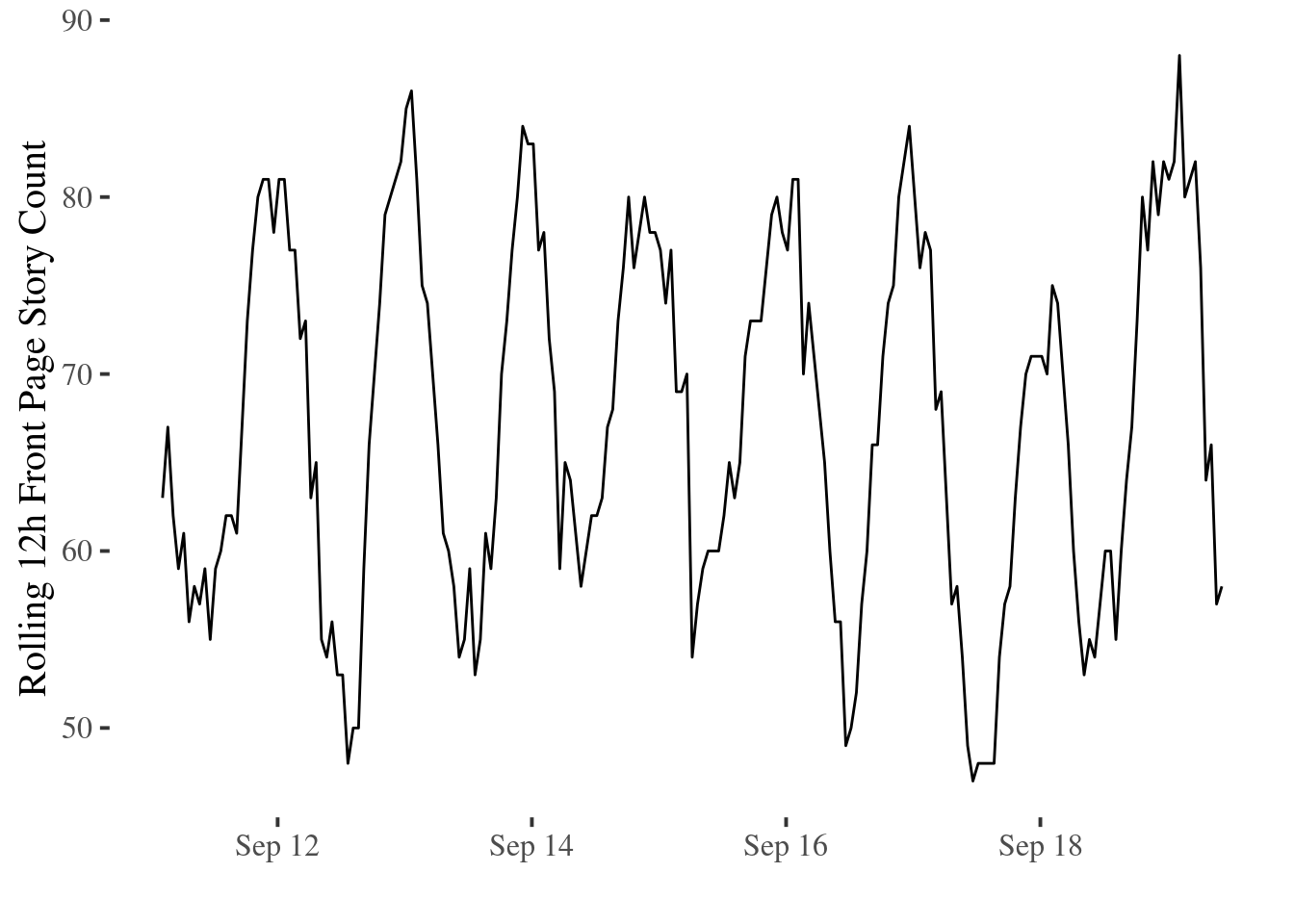This is an arbitrary slice of the data. Note the obvious cyclic pattern is pretty much by construction. Each story is counted by 12 sliding windows (x-axis), thus we have build-in autocorrelation in this series. Nonetheless, this is clear evidence of time-of-day effect.

Mean rolling story count by hour of day (UTC) also shows it:

```story_counts %>%

mutate(

hour_of_day = factor(

strftime(interval_begin, "%H", tz = "UTC")

)

) %>%

group_by(hour_of_day) %>%

summarise(story_counts_rolling_12h = mean(story_counts_rolling_12h, na.rm = T)) %>%

ggplot(., aes(x = hour_of_day, y = story_counts_rolling_12h)) +

geom_bar(stat = "identity") +

ylab("Story Count over Last 12h") + xlab("Hour of Day (UTC)") +

theme_tufte(base_size = 15)
```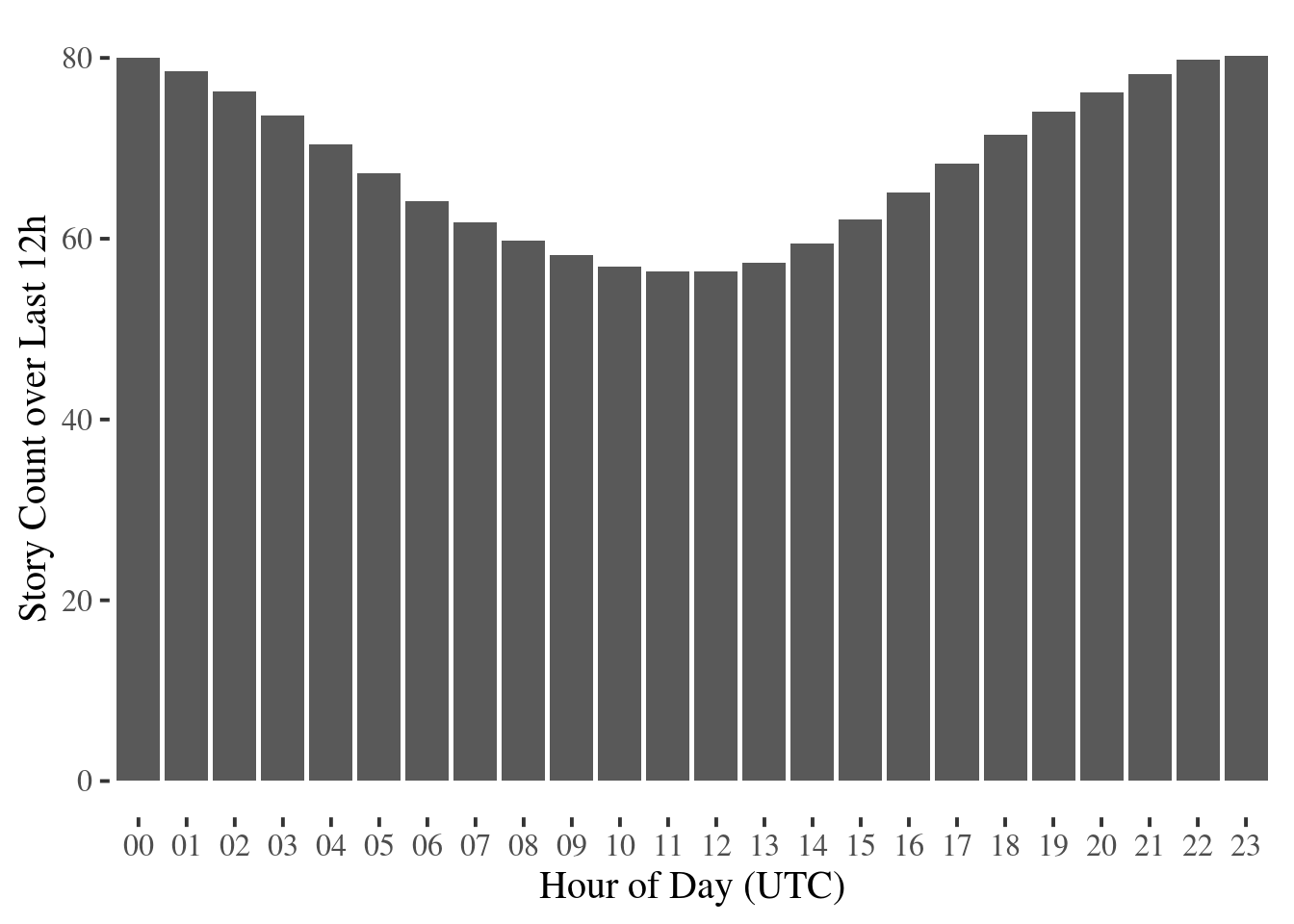Let’s pick the hour with highest story volume vs lowest volume, remove autocorrelation by choosing a larger time step, and plotting the entire dataset, the gap is quite visible.

```story_counts %>%

mutate(

hour_of_day = factor(

strftime(interval_begin, "%H", tz = "UTC")

)

) %>%

filter(hour_of_day == "11" | hour_of_day == "00") %>%

ggplot(aes(

x = interval_begin,

y = story_counts_rolling_12h,

linetype = hour_of_day

)) +

geom_line() +

ylab("Tiling Story Count over Last 12h") + xlab("") +

theme_tufte(base_size = 15)
```
``## Warning: Removed 1 rows containing missing values (geom_path).``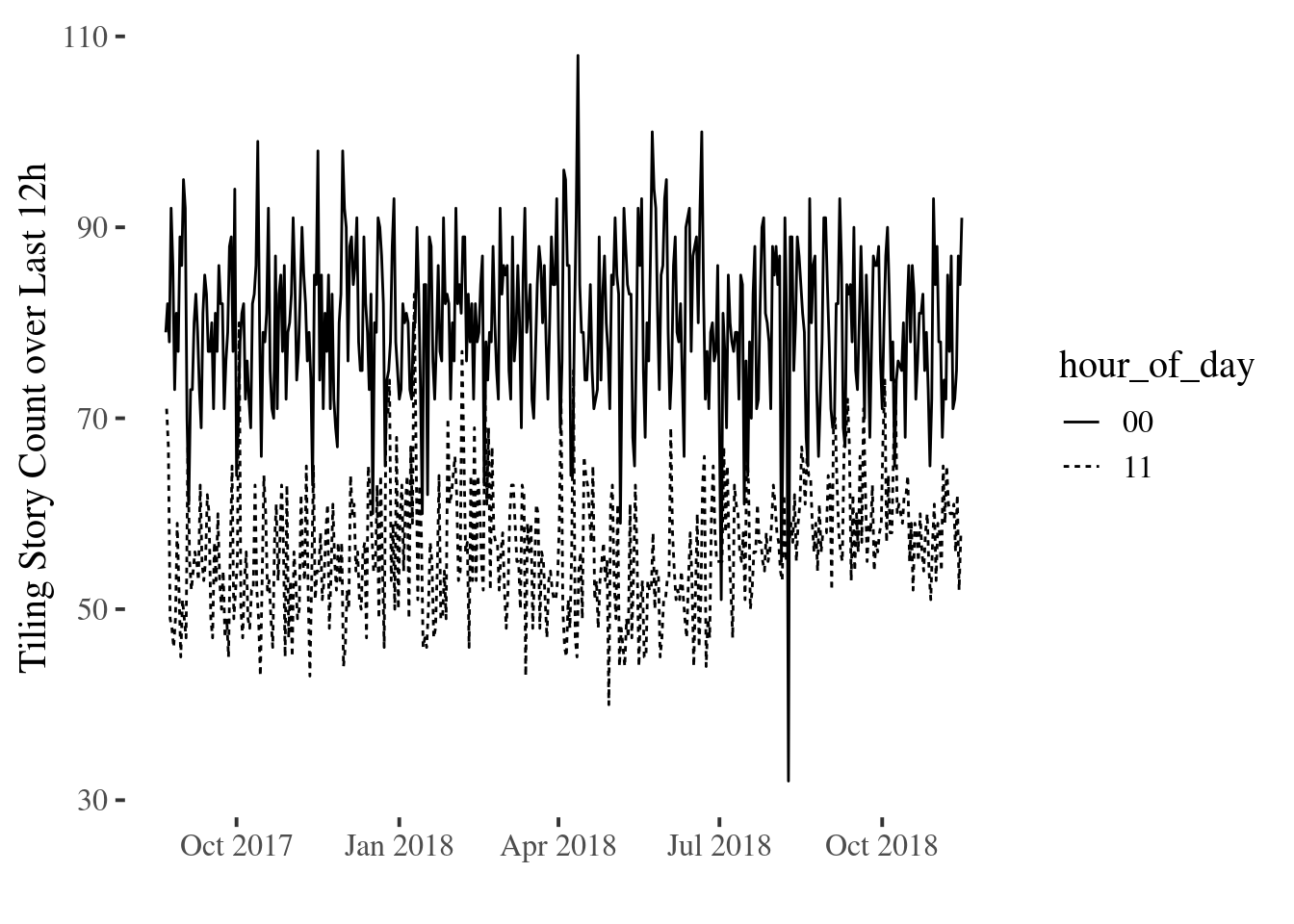```stories %>%

left_join(hnstats, by = c("story_id" = "story_id", "last_seen" = "interval_begin")) %>%

mutate(

(story_point_begin + story_point_delta) /

as.numeric(last_seen - created_at, units = "hours")

) -> stories_when_last_seen

stories_when_last_seen
```
```mean_sp <- mean(stories_when_last_seen\$lifetime_mean_hourly_point)

label = paste("Mean:", round(mean_sp, 2), sep=" ")

stories_when_last_seen %>%

geom_histogram(binwidth = 5) +

annotate("text", x=mean_sp + 22, y=7000, label=label) +

linetype="dashed") +

theme_tufte(base_size = 15)
```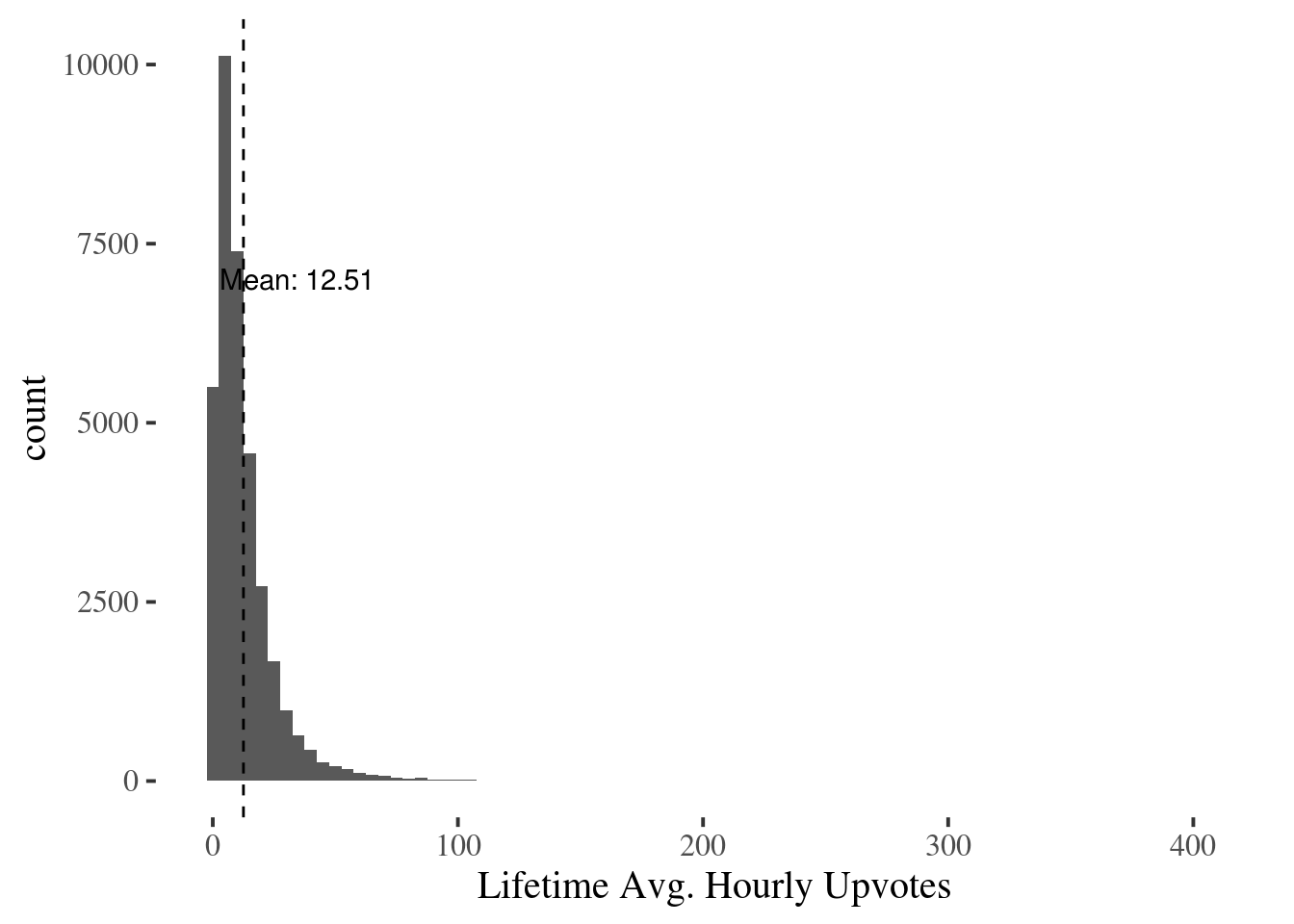```stories %>%

left_join(hnstats, by = c(

"story_id" = "story_id",

"first_seen" = "interval_begin"

)) -> stories_when_first_seen

stories_when_first_seen %>%

select(

story_id,

story_point_begin,

story_point_delta,

comment_count_begin,

comment_count_delta,

story_rank_begin,

story_rank_end

) %>%

inner_join(stories_when_last_seen, by = "story_id") -> stories_all

stories_all
```
```hours = str_pad(c(0:23), 2, pad="0")

stories_all %>%

mutate(

is_big_story= factor(frontpage_duration > as.difftime(12, units="hours")),

story_initial_age=as.numeric(first_seen - created_at, units="hours"),

story_initial_rank=as.factor(story_rank_begin.x),

hour_of_day=factor(

strftime(created_at, "%H", tz="UTC"))

) %>%

select(story_id,

is_big_story,

hour_of_day,

story_initial_rank,

story_initial_age,

story_point_begin.x,

comment_count_begin.x) -> comment_model_dataset
```
```set.seed(123)

train <- comment_model_dataset %>% sample_frac(.70)

test  <- anti_join(comment_model_dataset, train, by = 'story_id')
```
```stories_all %>%

mutate(frontpage_mean_hourly_point=(story_point_begin.y - story_point_begin.x) /

as.numeric(frontpage_duration, units="hours"),

pre_frontpage_mean_hourly_point=story_point_begin.x /

as.numeric(first_seen - created_at, units="hours")) %>%

gather(Phase, value, frontpage_mean_hourly_point:pre_frontpage_mean_hourly_point) -> mean_story_points

mean_story_points %>%

ggplot(., aes(x=value, y=stat(density))) +

geom_density(aes(linetype=Phase)) +

geom_hline(yintercept=0, colour="white", size=1) +

scale_linetype_discrete(

name="Phase",

breaks=c("frontpage_mean_hourly_point", "pre_frontpage_mean_hourly_point"),

labels=c("Front page", "New")) +

theme_tufte(base_size = 15)
```
``## Warning: Removed 3908 rows containing non-finite values (stat_density).``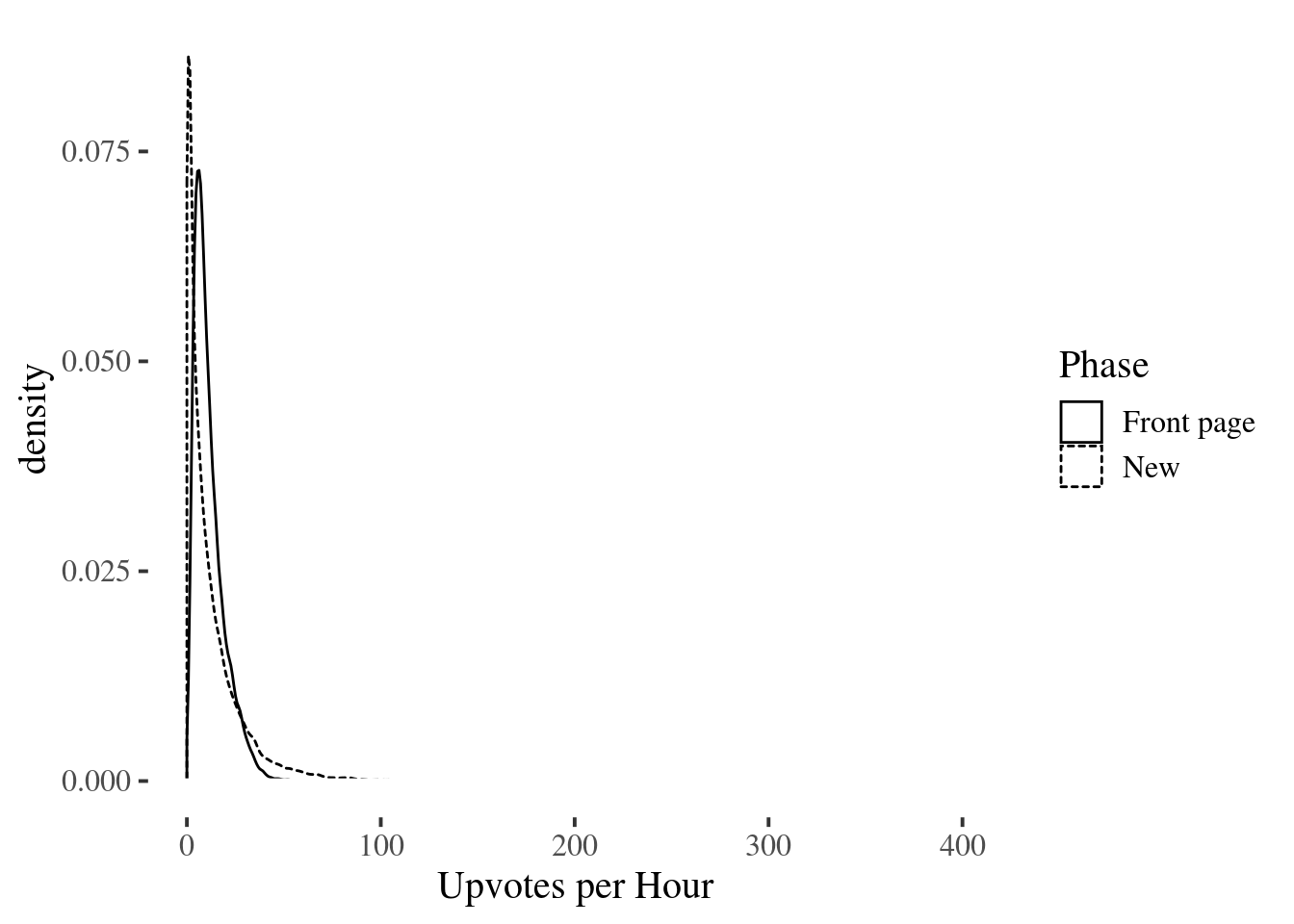```mean_story_points %>%

ggplot(., aes(x=log(value + 1), y=stat(density))) +

geom_histogram(color="white", aes(fill=Phase), alpha=0.2, bins=50) +

guides(fill=FALSE) +

geom_density(aes(linetype=Phase)) +

geom_hline(yintercept=0, colour="white", size=1) +

scale_linetype_discrete(

name="Phase",

breaks=c("frontpage_mean_hourly_point", "pre_frontpage_mean_hourly_point"),

labels=c("Front page", "New")) +

``## Warning: Removed 3908 rows containing non-finite values (stat_bin).``
``## Warning: Removed 3908 rows containing non-finite values (stat_density).``